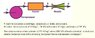• Koenigsegg17

## Homework Statement

See attachment please (Note: a lot of extra information is given, all of the information given for the compressor is possibly redundant).

## Homework Equations

1) SFEE (steady flow energy equation): q + W = (h2 - h1) + c2^2 - c1^1/2000 + g(z2 - z1)/1000

2) Isentropic relationship: T2/T1 = (p2/p1)^(y-1/y).. (where y = gamma = 1.4)

## The Attempt at a Solution

Apply the SFEE to find T2, but how?

I have tried the following:

q + W = (h2 - h1) + c2^2 - c1^1/2000 + g(z2 - z1)/1000.. where W = 0 (no shaft used), c2^2 - c1^1/2000 = 0 (no velocity information provided) and g(z2 - z1)/1000 = 0 (no change in height).

Therefore: q = (h2 - h1)
q = cp (T2 - T1).. where cp = 1.01kg/K, and cp (T2 - T1) is (h2 - h1) for an isobaric process.

Multiplying both sides of the equation by mass flow rate (so that the power given (i.e. heat) can be used in the equation).
q x m = heat energy [kW].

I get an answer of 5229.3 degrees C. (convert units to Kelvins during calculations).

•